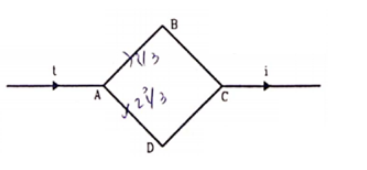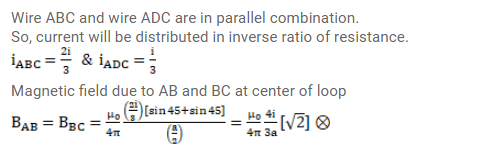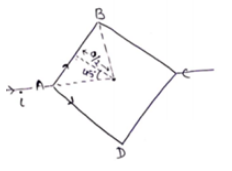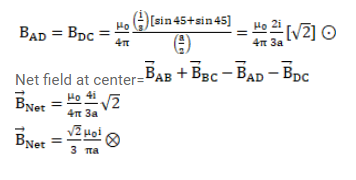# Figure shows a square loop A B C D

Question:

Figure shows a square loop $A B C D$ with edge length $a$. The resistance of the wire $A B C$ is $r$ and that of $A D C$ is $2 r$. Find the magnetic field $B$ at the center of the loop assuming uniform wires.Solution: